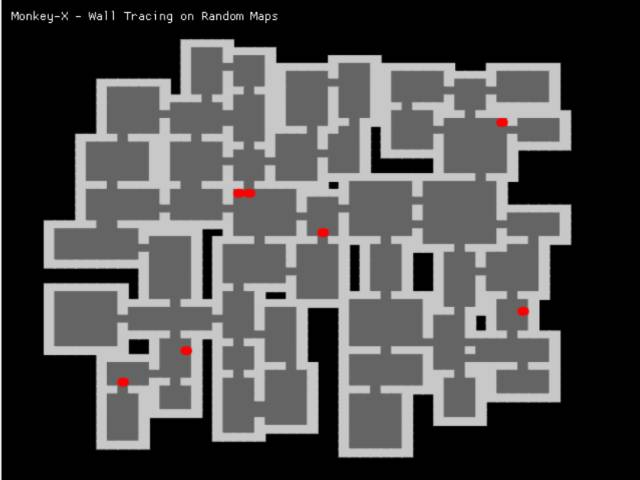-=+=- -=+=- -=+=- -=+=- -=+=- -=+=- -=+=- -=+=- -=+=- -=+=- -=+=- -=+=- -=+=- -=+=- -=+=- -=+=- -=+=- -=+=- -=+=- -=+=- -=+=- -=+=- -=+=- -=+=- -=+=- -=+=- -=+=- -=+=- -=+=- -=+=- (c) WidthPadding Industries 1987 0|456|0 -=+=- -=+=- -=+=- -=+=- -=+=- -=+=- -=+=- -=+=- -=+=- -=+=- -=+=- -=+=- -=+=- -=+=- -=+=- -=+=- -=+=- -=+=- -=+=- -=+=- -=+=- -=+=- -=+=- -=+=- -=+=- -=+=- -=+=- -=+=- -=+=- -=+=-
SoCoder -> Snippet Home -> Misc

PakzCreated : 20 February 2017
Edited : 20 February 2017
Language : Monkey

Wall Tracing on Random Maps (rpg)

From the book ai for game developersI finally started understanding more things from a book I bought a couple of years ago(ai for game developers) One part in the book explains how to do Wall Tracing.
From the Rogue basin and other sources I started learning and understanding how to make random levels for rpg's and such. I combined the two things.

The code below is MonkeyX1. It creates random levels filled with a random number of entities that crawl across the levels. Based on time it creates and displays a new level. (space can also be used to create a new level)
The size of the levels is also random.

Here the html 5 page :

cromdesi.home.xs4all.nl/html5/walltracing/MonkeyGame.html

Monday, 20 February 2017, 16:38
JayenkaiI'm glad you're learning all these little random-generation techniques.
I've found this kind of thing totally invaluable when doing AGameAWeek, as I rarely have the time to design levels, whilst cramming the game into such small spaces of time.
But even when working at a slower pace, it's still great to have this kind of randomisation within your games.

I'd love to see you tackle a nice big game, with this sort of thing.Monday, 20 February 2017, 17:41
PakzI hope to create bigger games one day. But I am still having to balance between learning all the things needed for making games.

Making good graphics is something that I am way behind with. So it will most likely take me years before I make something big.

I hope to do a couple of hours of #spritesomething today. (Drawing more river fish)Wednesday, 22 February 2017, 09:47
rskgamesHi Pakz,
I am a fan of your code snippets. Found the coding train youtube channel from your tweets.

Do you have this book https://www.ai-junkie.com/books/toc_pgaibe.html. Source code found in https://github.com/wangchen/Programming-Game-AI-by-Example-src

I have this book for the past 10 years, but not read it yet. Seeing your posts makes me want to read.

Thanks
Wednesday, 22 February 2017, 18:56
Pakz@rskgames.

I got your pm, I answered there..

I also checked (just yet) for that book in my archives. I still had it but end of last year the ebook company (mobipocket) stopped or bankrupted so I am not able to open the book file anymore(encryption). I contacted the book seller where I bought the book to see if they can help me read the book file. Not happy now!

I payed 50 euro's for that book! Paper is so much safer!Wednesday, 22 February 2017, 22:48
rskgamesSorry for the double post.